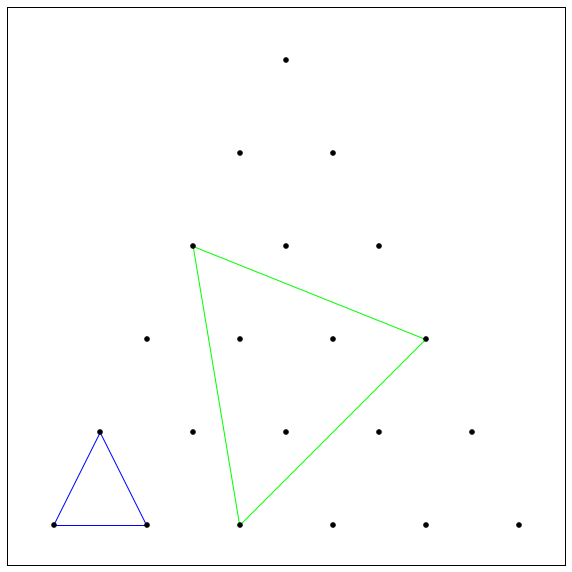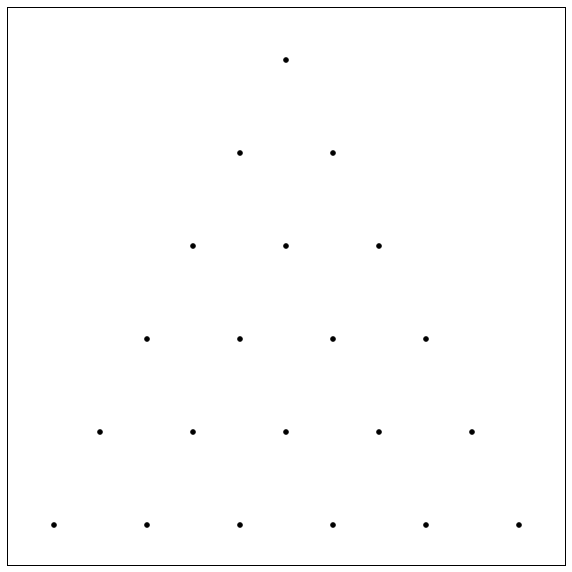# Too Many Triangles?

Probability Level 4How many equilateral triangles can you make by joining the dots with straight lines on this equilateral triangular lattice of perimeter length 6?Details and Assumptions:

• Assume the dots are in a perfect equilateral triangular lattice. My image is slightly stretched.

• The Two Triangles in the above image are both valid examples of unique Equilateral Triangles.

×